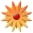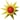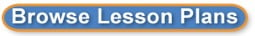Page Views: 7969

Email This Lesson Plan to Me
Overall Rating:(5.0 stars, 1 ratings)

 Subject(s): Grade 8 NETS-S Standard: Creativity and InnovationCommunication and CollaborationResearch and Information FluencyCritical Thinking, Problem Solving, and Decision MakingDigital CitizenshipTechnology Operations and ConceptsView Full Text of Standards School: Scoggins Middle School, Dallas, GA Planned By: angela bailey Original Author: angela bailey, Dallas
Transformations, Congruence, and Similarity
The three main Transformations are:
Rotation
Turn!
Reflection
Flip!
Translation
Slide!
After any of those transformations (turn, flip or slide), the shape still has the same size, area, angles and line lengths.
If one shape can become another using Turns, Flips and/or Slides, then the two shapes are called Congruent.
Resizing
The other important Transformation is Resizing (also called dilation, contraction, compression, enlargement or even expansion). The shape becomes bigger or smaller:
Resizing

If you have to resize to make one shape become another then the shapes are not congruent, but they are Similar.
MCC8.G.2 Understand that a two&#8208;dimensional figure is congruent to another if the second can be obtained from the first by a sequence of rotations, reflections, and translations; given two congruent figures, describe a sequence that exhibits the congruence between them. Standard-MCC8.G.3 Describe the effect of dilations, translations, rotations, and reflections on two&#8208;dimensional figures using coordinates.
 Week Objectives: Given a figure (pre-image) and a transformation specify the figure resulting from the transformation (image).
 Given a transformed figure (image) and a transformation, specify the original figure (pre-image).
 Given a figure (pre-image) and a transformation draw the result of the transformation.
 Given two figures, and a transformation recognize whether or not the second figure is the result of applying the transformation to the first figure.
 Given a figure (pre-image) and another figure that is a transformation of the first (image), describe the transformation that generates the second figure.
Pre-Assessment: Students will complete the online assessment from the site listed below: http://studyjams.scholastic.com/studyjams/jams/math/geometry/transformations.htm(on index card and turn in to your classroom basket)
Monday August 11th
Lessons vocabulary: Transformation, Translation, Rotation, and Reflection
Objective: What are the three Transformations?
What is a transformation? A transformation is a general term for four specific ways to manipulate the shape of a point, a line, or shape. The original shape of the object is called the pre-image and the final shape and position of the object is the image under the transformation.
Opening/Engage/I Do
Discuss with students their definition of a reflection, rotation, and translation. Show the following video to introduce the three transformations
https://search.yahoo.com/search;_ylt=A0LEVy4gIHlTlU0AkmBXNyoA?p=gangnam+style-transformation+style&fr2=sb-top&fr=yfp-t-430-s
Summary:
Students will write their definition/examples of the three transformations.
What is a rotation? A rotation is a transformation that turns a figure about a fixed point called the center of rotation. An object and its rotation are the same shape and size, but the figures may be turned in different directions. (Turn)
What is a reflection? A Reflection is a transformation in which the figure is the mirror image of the other. (Flip)

What is a translation? A translation is moving a shape, without rotating or flipping it. "Sliding" The shape still looks exactly the same, just in a different place. (Slide)
Work-time/Explore/We Do
Student work with their group members to complete the following interactive activity
Introduce the activity with the following interactive link: http://www.shodor.org/interactivate/activities/Transmographer/r>Reteach: Using the worksheet listed below, I will work with students who need additional assistance. http://www.superteacherworksheets.com/geometry/translation-rotation-reflection-1_TZQTQ.pdf
Closing/Explain/You
Do (Post-Assessment)
Have each student choose a figure and apply 2 transformations to it (noting what he or she did). Have students change places and try to determine how to undo each transformation.
Tuesday Aug 12th
Pre-Assessment: Translate, rotate, and reflect the given two-dimensional object on the coordinate plane. (Put your answer on index card and turn in to your classroom basket)
Objective: Student will translate, reflect, and rotate two-dimensional objects on the coordinate plane.
Opening/Engage/I Do (Identifying Transformations) Rotation (turn), reflection (flip-looking in the mirror), and translation (slide)
Summary: Students will write a postcard to a student that is our explaining how to translate, reflect, and rotate two-dimensional objects on the coordinate plane.
Work-time/Explore/We Do
Working with their group members, students will use the large coordinate grid to graph (Act out) transformations.
One person will choose a figure and the transformation. The other group members will stand on the points of intersections for each figure.
Reteach- (Small Group)-Review how to graph ordered pairs. Review the three transformations and how each move along the coordinate graph.
Closing/Explain/You Do (Post-Assessment)-Students will summarize their learning
Exit Pass (graph paper)
Make sure you label the points of the pre-image and the new image. Students will create one shape and use the three transformations to move the shape.
Wednesday Aug 13th
Pre-Assessment: To identify rotation of 90 degrees, 180 degrees, and 270 degrees, students are given a short matching assessment.
Objective: Students will rotate image 90 degrees, 180 degrees, and 270 degrees (clockwise and counter clockwise) and describe what happens to each point from the pre-image to the new image.
Rotation notes: http://www.mathsisfun.com/geometry/rotation.html

The demonstration below shows you how to easily perform the common Rotations (i.e. rotation by 90 , 180 , or rotation by 270). There is a neat 'trick' to doing these kinds of transformations. The basics steps are to graph the original point (the pre-image), then physically 'rotate' your graph paper, the new location of your point represents the coordinates of the image. It's much easier to understand these steps if you watch the visual demonstration below. (Click on each on the degrees 90, 180, and 270 to see the steps to rotate an image)
Opening/Engage
Show the video: http://www.brightstorm.com/math/geometry/transformations/rotations/
What three things you need to know to complete a rotation problem?
a. Point of rotation
b. Angle of rotation
c. Direction (clockwise or counter clockwise)
Demonstrate clockwise and counter clockwise
The distance from the center to any point on the shape stays the same.
Every point makes a circle around the center.
Summary: In their own words, students will write the steps to rotate image 90 degrees, 180 degrees, and 270 degrees (clockwise and counter clockwise) and describe what happens to each point from the pre-image to the new image.( \$2 Summaries- With each word worth 10 cents, write a \$2 summary of the learning from the lesson.
Teacher Note: This can be scaffold by giving students specific words related to the learning
that they must include in their summaries. This can be increased to any amount
of money.
Work-time/Explore/We Do
Students will work with their group to complete the rotation worksheet.
http://www.kutasoftware.com/FreeWorksheets/GeoWorksheets/12-Rotations.pdf
Reteach:
Small Group-Review 90, 180, 270 degree-model each rotation and check for understanding. Login to https://ple.platoweb.com and complete the rotation review lesson.
Closing/Explain/You Do-Summary
Key Points Summary-Students make a list of bulleted key points of the learning from the lesson.
Using the Smart board, a student from each group will model the steps to rotate an image 90 degrees, 180 degrees, and 270 degrees and what happened to each point from the pre-image to the new image.
Opening/Engage/I Do
Thursday Aug 14th
Pre-Assessment- Match the graph with rule: Reflect over the x axis, reflect over the y axis, and reflect across a certain line.
Objective: Students will graph each figure and its image under the given reflection. Find the coordinates of the vertices of each image. (Reflect over the x and y axis, and over a given line. Students will write the rule for the reflection)
Opening/Engage/I Do
Teacher will use the site below to introduce reflections. Students should take notes and the teacher will check for understanding after each section.
http://www.mathsisfun.com/geometry/reflection.html
Summary: Revisit Anticipation Guide/Pre-Assessment -Ask students to go back to the anticipation guide/pre-assessment from the beginning of the lesson and revise their answers. Ask them to justify the changes.
Work-time/Explore/We Do
Using Partner Puzzle
 Have students work in partners and use rulers and graph paper to create a shape on the top of the page. Partners should then exchange papers and draw the reflection of the figure on the bottom half of the paper.
Reteach- Using Mirror Image Small group instruction-additional assistance
Place a mirror where all children can see it. Choose a student to come up to the mirror and raise his right hand. Ask him which hand the mirror image seems to be raising. Choose different volunteers, and change the direction: Raise your left hand or Swing your right leg.
Closing/Explain-You Do/Summary
Ticket out the Door: Students will write a summary of the lesson to give to a student that is absent.
Friday Aug 9th
Friday is a make-up day/Extension of the weeks lesson/Reteach for those that didnt master the standard(s).
Monday and Tuesday should be completed:

 Materials: Whiteboards, CDs and DVDs, Hard Drives, Printers, Flash/USB Drives, Reading, Writing, Math, Middle